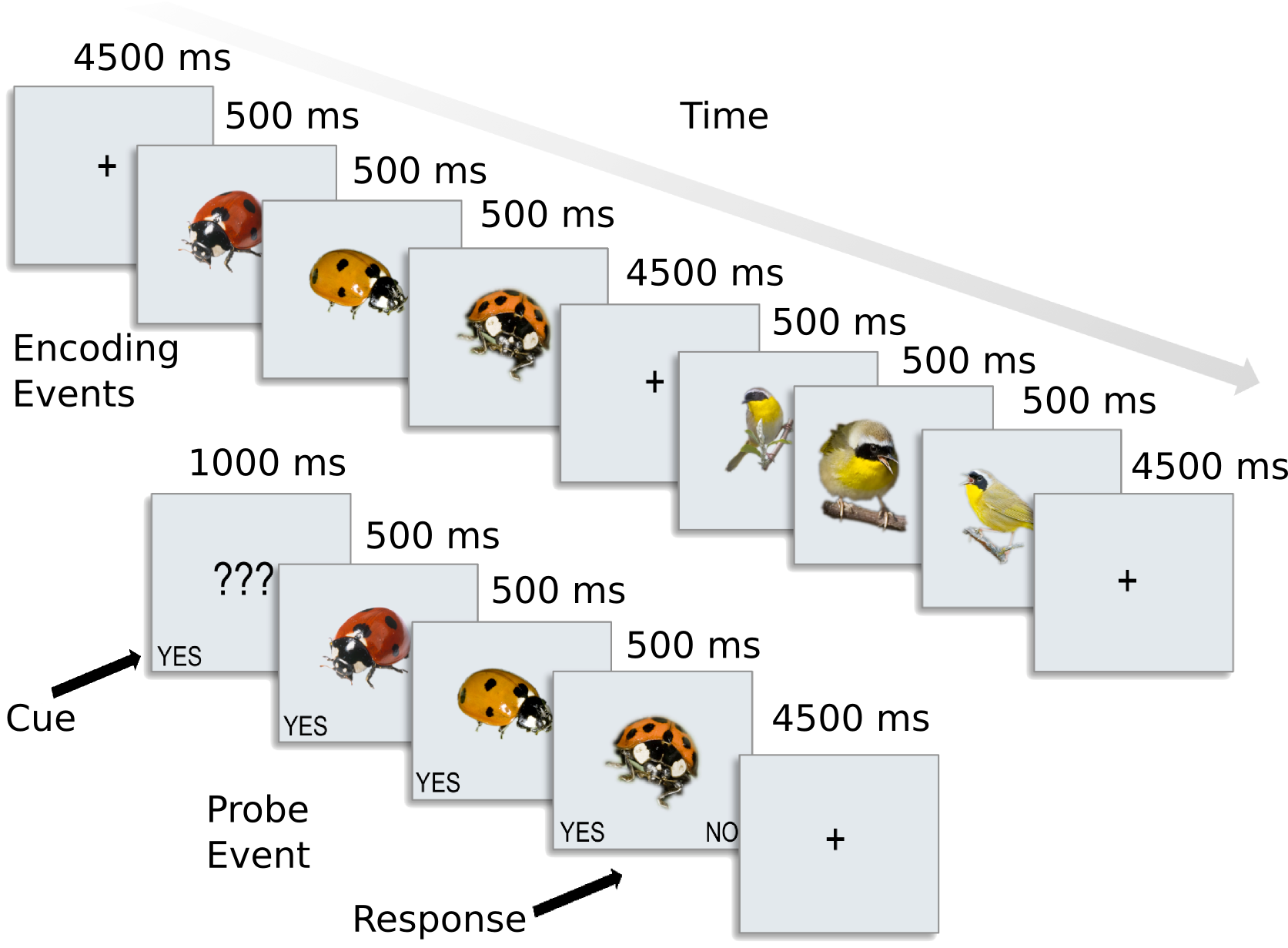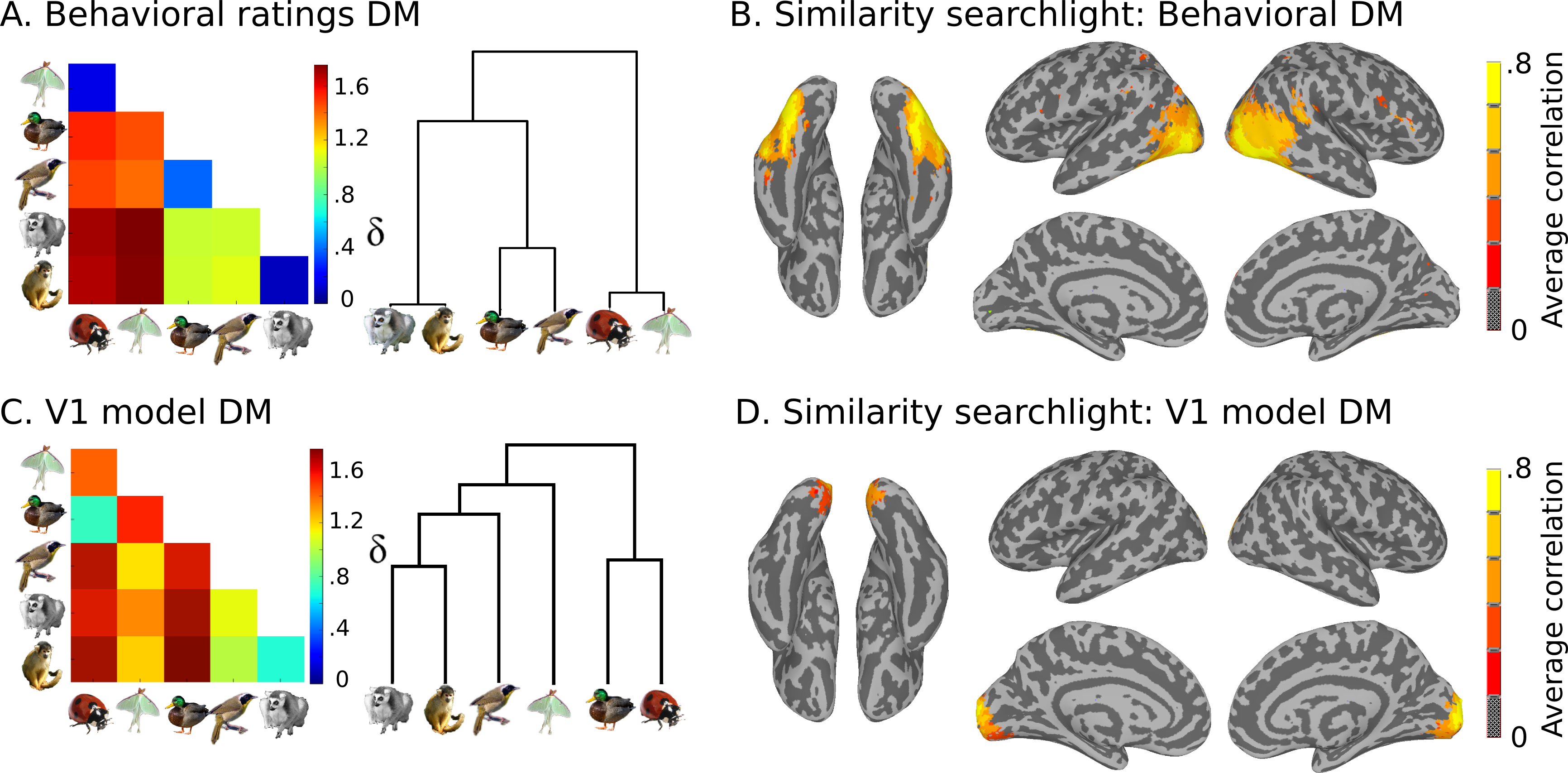# Introduction¶

## Goals of this course¶

• Describe the typical MVPA approaches (correlation analysis, classification analysis, representational similarity analysis applied to both regions of interest and across the whole brain in a searchlight approach) described in the literature.

• Learn how to use CoSMoMVPA to perform these analyses:
• Understand the dataset structure to represent both the data itself (e.g. raw measurements or summary statistics) and its attributes (e.g. labels of conditions (targets), data acquisition run (chunks).

• See how parts of the data can be selected using slicing and splitting, and combined using stacking

• Introduce measures that compute summaries of the data (such as correlation differences, classification accuracies, similarity to an a prior defined representational simillarity matrix) that can be applied to both a single ROI or in a searchlight.

• Make yourself an independent user, so that you can apply the techniques learnt here to your own datasets.

### Not covered in this course¶

• Preprocessing of fMRI data

• Learning to use Matlab / Octave

• Other dataset types than volumetric fMRI data (MEEG, surface-based fMRI)

• How to become a CoSMoMVPA developer

## Sample Dataset¶

The dataset used here contains preprocessed data for 8 subjects from [CGG+12]. In this experiment, participants were presented with categories of six animals: 2 primates: monkeys and lemurs; 2 birds: mallard ducks and yellow-throated warblers; and 2 bugs: ladybugs and luna moths.For each participant, the following data is present in the ak6 (for Animal Kingdom, 6 species) directory:

- s0[1-8]/                  This directory contains fMRI data from 8 of the 12
participants studied in the experiment reported in
Connolly et al. 2012 (Code-named 'AK6' for animal
kingdom, 6-species). Each subject's subdirectory
contains the following data:
- glm_T_stats_perrun.nii A 60-volume file of EPI-data preprocessed using
AFNI up to and including fitting a general linear
model using 3dDeconvolve. Each volume contains the
t-statistics for the estimated response to a one
of the 6 stimulus categories. These estimates were
calculated independently for each of the 10 runs
in the experiment.
- glm_T_stats_even.nii   Data derived from glm_T_stats_perrun.nii.
- glm_T_stats_odd.nii    Each is a 6-volume file with the T-values averaged
across even and odd runs for each category.
- brain.nii              Skull-stripped T1-weighted anatomical brain image.
that used in Connolly et al. 2012.


Also present are model similarity structures, which you can see here:This data is stored in the models directory:

- models
- behav_sim.mat          Matlab file with behavioural similarity ratings.
- v1_model.mat           Matlab file with similarity values based on
low-level visual properties of the stimuli.


## To cover in this course¶

• CoSMoMVPA concepts and dataset structure.

• Basic operations on datasets.

• Introduction to common MVPA measures:

• correlation difference

• classification accuracy

• representational similarity matching

• Common MVPA techniques:

• ROI analysis

• Searchlight analysis